# Question Solved1 AnswerLabel the following biological entities from smallest to largest, starting at 1. Chloroplast Testosterone Water molecule $$\mathrm{Na}+/ \mathrm{K}+$$ ATPase enzyme Virus Yeast cell Which of the following shows a hydrogen bond that could form between two of the molecules? Proteins contain several different types of bond. Say that we took a protein from a Label the following biological entities from smallest to largest, starting at 1. Chloroplast Testosterone Water molecule $$\mathrm{Na}+/ \mathrm{K}+$$ ATPase enzyme Virus Yeast cell Which of the following shows a hydrogen bond that could form between two of the molecules? Proteins contain several different types of bond. Say that we took a protein from a human body, heated it to $$100 \circ \mathrm{C}$$, and took measurements from it periodically to see which bonds are broken. Which of these bonds would be most likely to remain intact the longest? Choose the best response. Covalent bond between two neighboring amino acids Hydrogen bond among the backbone of a subunit Ionic bond between amino acid sidechains Hydrophobic attraction within a subunit If two atoms share a bonding electron pair fairly equally, the bond between these atoms is said to be: Polar covalent Nonpolar covalent Ionic Hydrogen bond This molecule is ethanol: Based on the location of the atoms, in which bonds would electrons be shared equally? select all that apply On the bond between $$\mathrm{C}-\mathrm{H}$$, the electrons would be shared equally On the bond between C-O, the electrons would be shared equally On the bond between $$\mathrm{O}-\mathrm{H}$$, the electrons would be shared equally On the bond between C-C, the electrons would be shared equally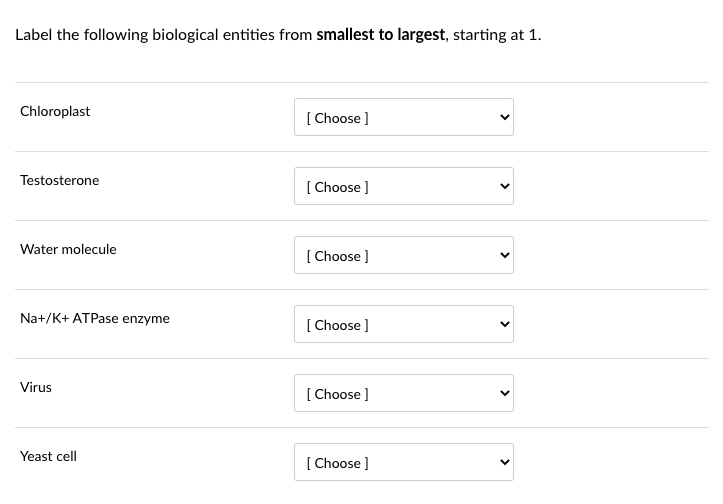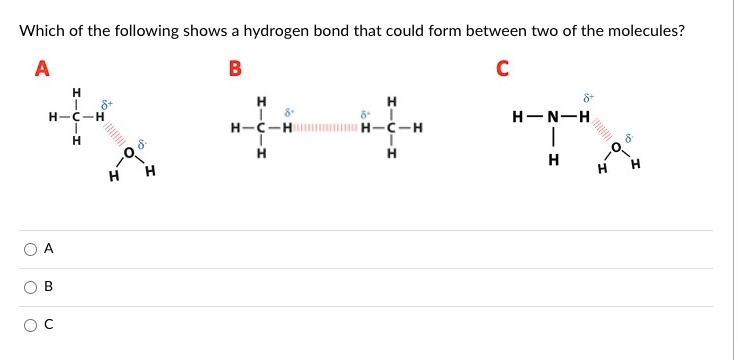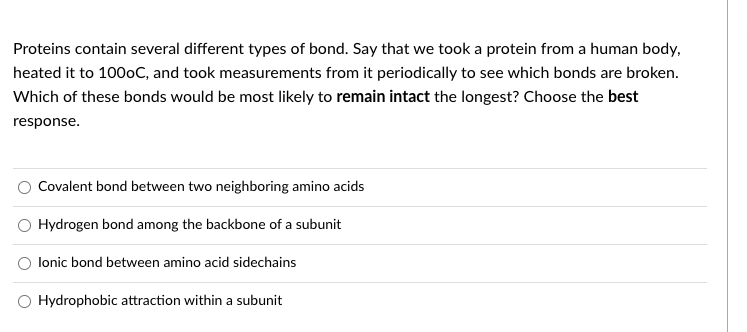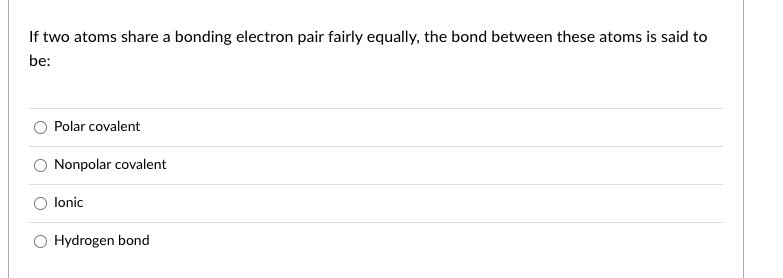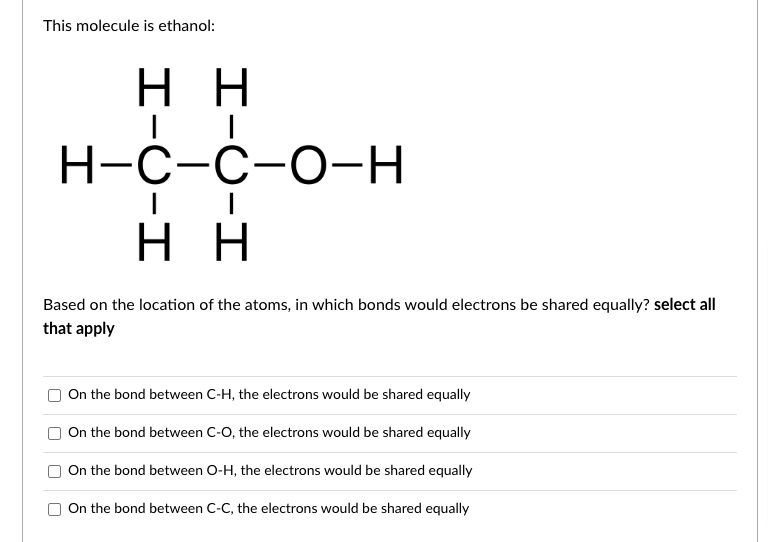pls only answer if u can do all questions thank u so much

Transcribed Image Text: Label the following biological entities from smallest to largest, starting at 1. Chloroplast Testosterone Water molecule $$\mathrm{Na}+/ \mathrm{K}+$$ ATPase enzyme Virus Yeast cell Which of the following shows a hydrogen bond that could form between two of the molecules? Proteins contain several different types of bond. Say that we took a protein from a human body, heated it to $$100 \circ \mathrm{C}$$, and took measurements from it periodically to see which bonds are broken. Which of these bonds would be most likely to remain intact the longest? Choose the best response. Covalent bond between two neighboring amino acids Hydrogen bond among the backbone of a subunit Ionic bond between amino acid sidechains Hydrophobic attraction within a subunit If two atoms share a bonding electron pair fairly equally, the bond between these atoms is said to be: Polar covalent Nonpolar covalent Ionic Hydrogen bond This molecule is ethanol: Based on the location of the atoms, in which bonds would electrons be shared equally? select all that apply On the bond between $$\mathrm{C}-\mathrm{H}$$, the electrons would be shared equally On the bond between C-O, the electrons would be shared equally On the bond between $$\mathrm{O}-\mathrm{H}$$, the electrons would be shared equally On the bond between C-C, the electrons would be shared equally
More
Transcribed Image Text: Label the following biological entities from smallest to largest, starting at 1. Chloroplast Testosterone Water molecule $$\mathrm{Na}+/ \mathrm{K}+$$ ATPase enzyme Virus Yeast cell Which of the following shows a hydrogen bond that could form between two of the molecules? Proteins contain several different types of bond. Say that we took a protein from a human body, heated it to $$100 \circ \mathrm{C}$$, and took measurements from it periodically to see which bonds are broken. Which of these bonds would be most likely to remain intact the longest? Choose the best response. Covalent bond between two neighboring amino acids Hydrogen bond among the backbone of a subunit Ionic bond between amino acid sidechains Hydrophobic attraction within a subunit If two atoms share a bonding electron pair fairly equally, the bond between these atoms is said to be: Polar covalent Nonpolar covalent Ionic Hydrogen bond This molecule is ethanol: Based on the location of the atoms, in which bonds would electrons be shared equally? select all that apply On the bond between $$\mathrm{C}-\mathrm{H}$$, the electrons would be shared equally On the bond between C-O, the electrons would be shared equally On the bond between $$\mathrm{O}-\mathrm{H}$$, the electrons would be shared equally On the bond between C-C, the electrons would be shared equally